# Cycloconverters – Types & Applications

In industrial applications, two forms of electrical energy Direct Current (DC) and Alternate current (AC) are used. Constant voltage and constant current AC is directly available. However, for different applications, different forms, different voltages, and/or different currents are needed. Converters are needed to achieve different forms. These converters are classified as rectifiers, choppers, inverters, and cyclo converters.

A cycloconverter is a device that converts AC, power at one frequency into AC power of an adjustable but lower frequency without any direct current, or DC, stage in between. It can likewise be acknowledged as a static recurrence charger and holds silicon-regulated rectifiers. Cyclo-converters are used in very large variable frequency drives with ratings from few megawatts up to many tens of megawatts.

The principle of the cyclo-converter is described below by using single-phase to single-phase cyclo-converter.

A single phase input cycloconverter is shown below (a) 50 Hz, (b) 25Hz, (c) 12.5 Hz single-phase input to single-phase output cycloconverter is shown below.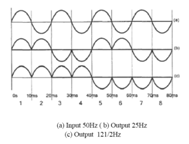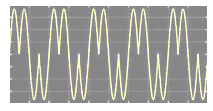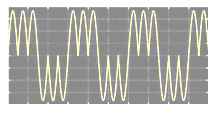Rectifier converts from Single-phase or three-phase AC to variable dc Voltage. Choppers convert from DC to variable dc voltage. Inverters convert from DC to variable magnitude variable frequency single-phase or three-phase AC. Cyclic converters convert from single-phase or three-phase AC to variable magnitude variable frequency single-phase or three-phase AC. A cycloconverter is having four thyristors divided into a positive and negative bank of two thyristors each.

### Cycloconverter Basic Schematic:

The cycloconverter is connected to input between 30 and 31 as shown below. The motor is connected between 25& 26.

Depending upon the triggering pulses fed to a set of 8 SCRs between their gate and cathode we get F or F/2 or F/3.

### Types of Cycloconverters:

There are mainly two types of cyclo converters blocking mode type and circulating mode type. When the load current is positive, the positive converter supplies the required voltage, and the negative converter is blocked. Suppose if the load current is negative, then the negative converter supplies the voltage and the positive converter is blocked. This operation is called blocking mode operation. The cyclo converters which are using this method are called blocking mode cyclo converters.

By chance, if both converters are enabled, then the supply will be short-circuited. To avoid this, an intergroup reactor (IGR) must be connected between the converters. If both the converters are enabled, then a circulating current is produced. This is unidirectional because the thyristors allow the current to flow in only one direction. The cyclo converters using this approach are called circulating current converters.

Blocking Mode Cycloconverters:

Blocking mode cyclo converters don’t need any intergroup reactor (IGR). Depends on the polarity, one of the converters is enabled. The blocking mode operation has some advantages and disadvantages over circulating mode operation. They don’t need any reactors hence size and cost is less. Only one converter is in conduction at all times rather than two. During delay time current stays at zero distorting the voltage and current waveforms. This distortion means complex harmonic patterns.

Circulating Current Cycloconverters:

Both the converters operate at all times in this case. The big disadvantage is an IGR is needed. The number of devices connecting to this is twice than of blocking current cycloconverter.

### Principles of Cycloconverters:

The operation principles of cyclo converters can be classified into the following three types based on the type of input AC supply applied to the circuit.

Single-Phase to Single-Phase Cycloconverter: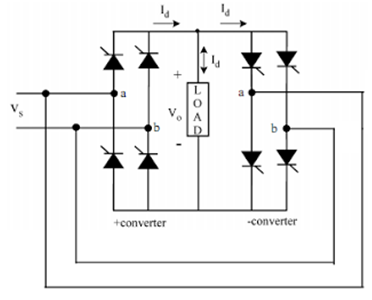Understanding of operation principles of cyclo converters should begin with single-phase to single-phase cycloconverter. This converter is having back to back connection of two full-wave rectifiers. Suppose for getting one-fourth of input voltage at the output, for the first two cycles of Vs the positive converter operates supplying current to the load and it rectifies the input voltage. In the next two cycles, the negative converter operates supplying current in the reverse direction. When one of the converters operates the other one is disabled so that there is no current circulating between rectifiers. In the below figure Vs represents input supply voltage and Vo is the required output voltage which is one-fourth of the supply voltage.

Image for One-fourth of input voltage at the output using 1-phase to 1-phase Cycloconverter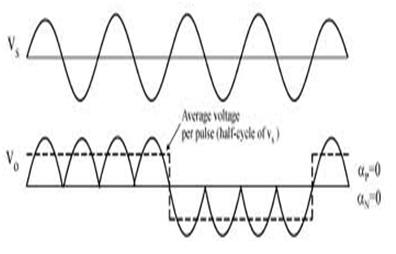Three-Phase to Single-Phase Cycloconverters:

Like as above converters, three-phase to single-phase cycloconverter applies rectified voltage to the load. Positive Cycloconverters will supply positive current only while negative converters will supply negative current only. The cyclo converters can operate in four quadrants as (+v, +i), (+v, -i) rectification modes and (-v, +i), (-v, -i) inverting modes. The polarity of the current determines if the positive or negative converter should be supplying power to the load. When there is a change in current polarity, the converter previously supplying current is disabled and the other one is enabled. During the current polarity reversal, the average voltage supplied by both the converters should be equal.

Three-Phase to Three-Phase Cycloconverter:

Two basic configurations are available for three-phase cyclo converters such as delta and wye. If the outputs of the above converter are connected in wye or delta and if the output voltages are 120º phase-shifted the resulting converter is three-phase to the three-phase converter. The three-phase converters are mainly used in machine drive systems running three-phase synchronous and induction machines.

### Applications of Cycloconverters:

Cycloconverters can produce harmonic rich output voltages. When cyclo converters are using for a running AC machine, the leakage inductance of the machine filters most of the high-frequency harmonics and reducing the voltage of the lower order harmonics.

Controlling the Speed of the Single Phase Induction Motor

Single-phase induction motors are widely used in many applications. Improvements in its performance mean a great saving in electrical energy consumption. A speed controller based on cycloconverter is proposed.